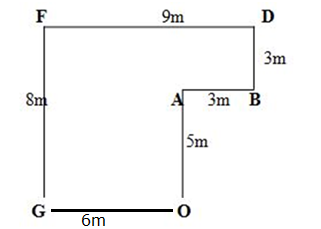# RRB Clerk Mains Reasoning (Day-03)

## Reasoning Materials for RRB Clerk Prelims:

Dear Aspirants, Our IBPS Guide team is providing new series of Reasoning Questions for RRB Clerk Mains 2020 so the aspirants can practice it on a daily basis. These questions are framed by our skilled experts after understanding your needs thoroughly. Aspirants can practice these new series questions daily to familiarize with the exact exam pattern and make your preparation effective.

Start Quiz

Condition based number series

Direction (1-5): The following questions are based on the number series given below.

1 3 4 5 7 8 1 2 1 8 3 4 7 5 1 8 3 4 5 9  8 1 7 4 5 8 9 5 9 7 1 6 9

Conditions:

i)Each odd prime number in the above series is replaced by the number which follows that odd prime number in the decimal number system.

ii)Each even prime number in the above series is replaced by the number which precedes that even prime number in the decimal number system.

iii) Each odd perfect square number in the above series is replaced by the number which is the square root of that number in the decimal number system.

Conditions are applied from (i) to (iii)

After applying the above conditions on the series answer the questions given below.

1) How many even numbers are there in the sequence each of which is/are immediately followed by an odd number in the final series?

A) None

B) Three

C) More than Three

D) Two

E) One

2) Which of the following digit is exactly between the one, which is 16th from the left end and which is 12th from the right end in the final series?

A) 6

B) 8

C) 4

D) 2

E) 3

3) Which of the following digit is 10th to the right of the one which is 14th from the left end in the final series?

A) 6

B) 8

C) 3

D) 4

E) 1

4) How many 6’s are there in the final series?

A) Two

B) Four

C) Six

D) One

E) Three

5) Which of the following digit is 12th  to the left of the one which is 8th  from the right end in the final series?

A) 6

B) 8

C) 3

D) 4

E) 1

Coded direction sense

Directions (6 – 10): Read the information given below carefully and answer the following questions. The data given are in some coded format, throughout and proceed further.

\$ – North                               @ – Either 5 meters or 9 meters

# – South                                © – Either 3 metres or 8 metres

% – West

& – East

i.e., P \$ Q means Point P is to the North of point Q.

P @ Q means Point P is either 5 metres or 9 metres from point Q

P \$& Q means Point P is to the North-East of point Q.

P \$© Q means Point P is North of point Q and in either 3 metres or 8 metres.

Point A is 5 metres north of point O, which is 6 metres away from point G.

I). B & A

IV). F %@ D

V). F \$% O

VII). G % O

Note: No two points at the same place.

6) If point X is 3 metre north of point A. then what will be the distance between points F and X?

A) 3m

B) 6m

C) 9m

D) 4.5m

E) None of these

7) If Y # G and with distance, then what will be the answer from the following?

A) Y \$& F

B) Y \$& B

C) A \$% Y

D) O \$& Y

E) None of these

8) What is the distance and direction of point A with respect to point F?

A) 9m, #%

B) 4√5m, \$&

C) 3√5m, #&

D) 3√5m, \$%

E) None of these

9) If Z #© F, then which of the following is true?

A) A & Z with 6m

B) Z & A with 9m

C) Z # B with 12m

D) B # Z with 3m

E) None of these

10) What is the shortest distance between point O and point F?

A) 9m

B) 3√8m

C) 10m

D) 4√5m

E) None of these

Directions (1-5):

Step-I: 1 4 4 6 8 8 1 2 1 8 4 4 8 6 1 8 4 4 6 9 8 1 8 4 6 8 9 6 9 8 1 6 9

Step-II: 1 4 4 6 8 8 1 1 1 8 4 4 8 6 1 8 4 4 6 9 8 1 8 4 6 8 9 6 9 8 1 6 9

Step-III: 1 4 4 6 8 8 1 1 1 8 4 4 8 6 1 8 4 4 6 3  8 1 8 4 6 8 3 6 3 8 1 6 3

The final series is as follows:

1 4 4 6 8 8 1 1 1 8 4 4 8 6 1 8 4 4 6 3  8 1 8 4 6 8 3 6 3 8 1 6 3

Directions (6-10):